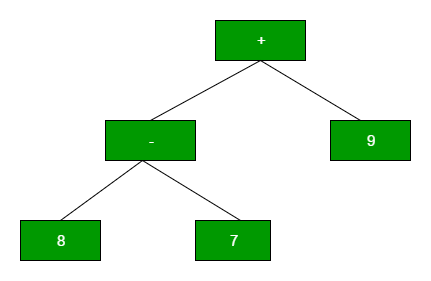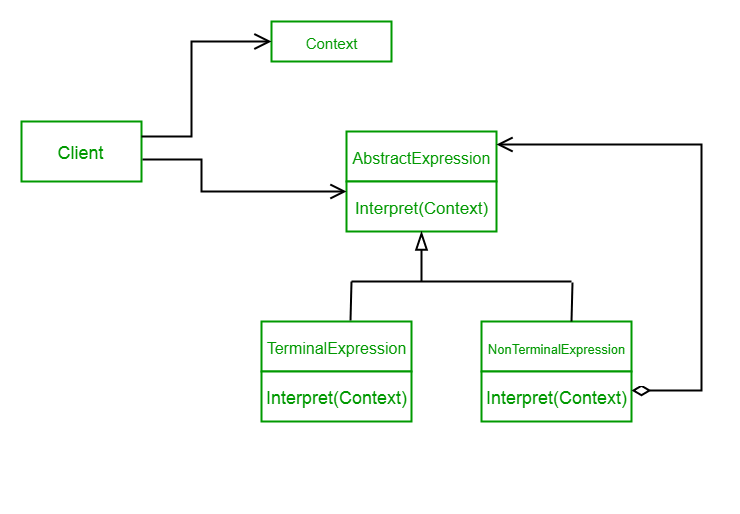Related Articles
Interpreter Design Pattern
• Last Updated : 22 Feb, 2018

Interpreter design pattern is one of the behavioral design pattern. Interpreter pattern is used to defines a grammatical representation for a language and provides an interpreter to deal with this grammar.

• This pattern involves implementing an expression interface which tells to interpret a particular context. This pattern is used in SQL parsing, symbol processing engine etc.
• This pattern performs upon a hierarchy of expressions. Each expression here is a terminal or non-terminal.
• The tree structure of Interpreter design pattern is somewhat similar to that defined by the composite design pattern with terminal expressions being leaf objects and non-terminal expressions being composites.
• The tree contains the expressions to be evaluated and is usually generated by a parser. The parser itself is not a part of the interpreter pattern.

For Example :
Here is the hierarchy of expressions for “+ – 9 8 7” :Implementing the Interpreter Pattern

UML Diagram Interpreter Design PatternDesign components

• AbstractExpression (Expression): Declares an interpret() operation that all nodes (terminal and nonterminal) in the AST overrides.
• TerminalExpression (NumberExpression): Implements the interpret() operation for terminal expressions.
• NonterminalExpression (AdditionExpression, SubtractionExpression, and MultiplicationExpression): Implements the interpret() operation for all nonterminal expressions.
• Context (String): Contains information that is global to the interpreter. It is this String expression with the Postfix notation that has to be interpreted and parsed.
• Client (ExpressionParser): Builds (or is provided) the AST assembled from TerminalExpression and NonTerminalExpression. The Client invokes the interpret() operation.

Let’s see an example of Interpreter Design Pattern.

 `// Expression interface used to ` `// check the interpreter. ` `interface` `Expression ` `{ ` `    ``boolean` `interpreter(String con); ` `} ` ` `  `// TerminalExpression class implementing ` `// the above interface. This interpreter  ` `// just check if the data is same as the  ` `// interpreter data. ` `class` `TerminalExpression ``implements` `Expression  ` `{ ` `    ``String data; ` ` `  `    ``public` `TerminalExpression(String data) ` `    ``{ ` `        ``this``.data = data;  ` `    ``} ` ` `  `    ``public` `boolean` `interpreter(String con)  ` `    ``{ ` `        ``if``(con.contains(data)) ` `        ``{ ` `            ``return` `true``; ` `        ``} ` `        ``else` `        ``{ ` `            ``return` `false``;   ` `        ``} ` `    ``} ` `} ` `// OrExpression class implementing ` `// the above interface. This interpreter  ` `// just returns the or condition of the  ` `// data is same as the interpreter data. ` `class` `OrExpression ``implements` `Expression  ` `{ ` `    ``Expression expr1; ` `    ``Expression expr2; ` ` `  `    ``public` `OrExpression(Expression expr1, Expression expr2)  ` `    ``{ ` `        ``this``.expr1 = expr1; ` `        ``this``.expr2 = expr2; ` `    ``} ` `    ``public` `boolean` `interpreter(String con)  ` `    ``{         ` `        ``return` `expr1.interpreter(con) || expr2.interpreter(con); ` `    ``} ` `} ` ` `  `// AndExpression class implementing ` `// the above interface. This interpreter  ` `// just returns the And condition of the  ` `// data is same as the interpreter data. ` `class` `AndExpression ``implements` `Expression  ` `{ ` `    ``Expression expr1; ` `    ``Expression expr2; ` ` `  `    ``public` `AndExpression(Expression expr1, Expression expr2)  ` `    ``{  ` `        ``this``.expr1 = expr1; ` `        ``this``.expr2 = expr2; ` `    ``} ` `    ``public` `boolean` `interpreter(String con)  ` `    ``{         ` `        ``return` `expr1.interpreter(con) && expr2.interpreter(con); ` `    ``} ` `} ` ` `  `// Driver class ` `class` `InterpreterPattern ` `{ ` ` `  `    ``public` `static` `void` `main(String[] args)  ` `    ``{ ` `        ``Expression person1 = ``new` `TerminalExpression(``"Kushagra"``); ` `        ``Expression person2 = ``new` `TerminalExpression(``"Lokesh"``); ` `        ``Expression isSingle = ``new` `OrExpression(person1, person2); ` `         `  `        ``Expression vikram = ``new` `TerminalExpression(``"Vikram"``); ` `        ``Expression committed = ``new` `TerminalExpression(``"Committed"``); ` `        ``Expression isCommitted = ``new` `AndExpression(vikram, committed);     ` ` `  `        ``System.out.println(isSingle.interpreter(``"Kushagra"``)); ` `        ``System.out.println(isSingle.interpreter(``"Lokesh"``)); ` `        ``System.out.println(isSingle.interpreter(``"Achint"``)); ` `         `  `        ``System.out.println(isCommitted.interpreter(``"Committed, Vikram"``)); ` `        ``System.out.println(isCommitted.interpreter(``"Single, Vikram"``)); ` ` `  `    ``} ` `} `

Output:

```true
true
false
true
false
```

In the above code , We are creating an interface Expression and concrete classes implementing the Expression interface. A class TerminalExpression is defined which acts as a main interpreter and other classes OrExpression, AndExpression are used to create combinational expressions.

• It’s easy to change and extend the grammar. Because the pattern uses classes to represent grammar rules, you can use inheritance to change or extend the grammar. Existing expressions can be modified incrementally, and new expressions can be defined as variations on old ones.
• Implementing the grammar is easy, too. Classes defining nodes in the abstract syntax tree have similar implementations. These classes are easy to write, and often their generation can be automated with a compiler or parser generator.

• Complex grammars are hard to maintain. The Interpreter pattern defines at least one class for every rule in the grammar. Hence grammars containing many rules can be hard to manage and maintain.

This article is contributed by Saket Kumar. If you like GeeksforGeeks and would like to contribute, you can also write an article using contribute.geeksforgeeks.org or mail your article to contribute@geeksforgeeks.org. See your article appearing on the GeeksforGeeks main page and help other Geeks.